Next: Large-Scale Microscopic Nuclear Mass Up: Nuclear Energy Density Functional Previous: The Energy Density Functional

## Variational Equations

By varying the energy functional (3) with respect to the density matrices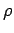and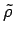one arrives at the HFB equations: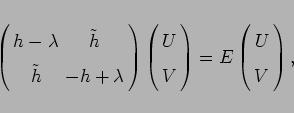(6)

where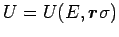,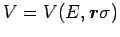are the HFB wave functions, and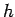and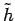are the local particle and pairing mean-field Hamiltonians.

The HFB equations (6), also called the Bogoliubov de Gennes equations by condensed matter physicists, are the generalized Kohn Sham equations of the DFT. It is worth noting that - in its original formulation  - the DFT formalism implicitly includes the full correlation functional. In most nuclear applications, however, the correlation corrections are added afterwards. Those corrections usually include the following terms: the center-of-mass correction, rotational correction associated with the spontaneous breaking of rotational symmetry, vibrational correction (quantum zero-point vibrational fluctuations), particle-number correction due to the broken gauge invariance, as well as other terms.

The spectrum of quasi-particle energies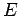is continuous for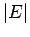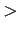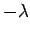and discrete for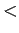. However, when solving the HFB equations on a coordinate-space lattice of points or by expanding quasi-particle wave functions in a finite basis, the quasi-particle spectrum is discretized and one can use the notation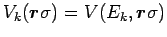and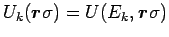. Since for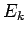0 and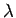0 the lower components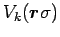are localized functions of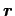, the density matrices,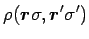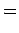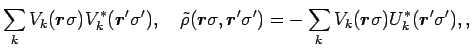(7)

are always localized. The norms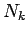of the lower components define the total number of particles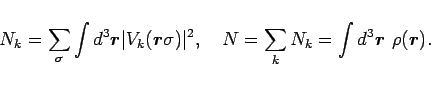(8)

For spherical nuclei, the self-consistent HFB equations are best solved in the coordinate space where they form a set of 1D radial differential equations [16,17]. In the case of deformed nuclei, however, the solution of deformed HFB equations in coordinate space is a difficult and time-consuming task. For axial nuclei, the corresponding 2D differential equations can be solved by using the basis-spline methods (see, e.g., Ref. ). For triaxial nuclei, 3D solutions in a restricted space are possible by using the so-called two-basis method .Next: Large-Scale Microscopic Nuclear Mass Up: Nuclear Energy Density Functional Previous: The Energy Density Functional
Jacek Dobaczewski 2006-01-17